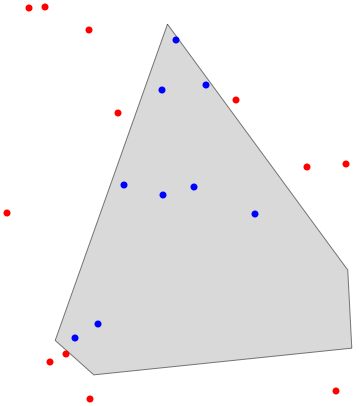# Implementing a fast point-in-polygon algorithm in MathematicaJust spend an enjoyable half hour reading about ingenious solutions to the “point in polygon” question, looking for a Mathematica implementation. Since I didn’t find any, I’ll post my port.

pnPoly::usage = "
pnPoly[{testx,testy},{{x1,y1},{x2,y2},...}] return true if {testx,testy} in inside polygon.
Polygon can be closed or not. A point will be inside exactly one member of a polygonal partitioning.
C-code by W. Randolph Franklin (http://www.ecse.rpi.edu/Homepages/wrf/Research/Short_Notes/pnpoly.html).
J. Wesenberg, 2008";
pnPoly[{testx_, testy_}, pts_List] := Xor @@ ((
Xor[#[[1, 2]] > testy, #[[2, 2]] > testy] &&
((testx - #[[2, 1]]) < (#[[1, 1]] - #[[2, 1]]) (testy - #[[2, 2]])/(#[[1, 2]] - #[[2, 2]]))
) & /@ Partition[pts, 2, 1, {2, 2}])


A test for the non-believers:

Needs["ComputationalGeometry"]
pol = (#[[ConvexHull[#]]]) &[RandomReal[{0, 1}, {12, 2}]];
Graphics[{PointSize[Large],
{FaceForm[LightGray], EdgeForm[Black], Polygon[pol]},
If[pnPoly[#, pol], {Blue, Point[#]}, {Red, Point[#]}] & /@
RandomReal[{0, 1}, {21, 2}]
}]
Clear[pol]


Original. The code is ported from this C-version by W.R. Franklin. Note that the Mathematica code is even shorter :)

int pnpoly(int nvert, float *vertx, float *verty, float testx, float testy)
{
int i, j, c = 0;
for (i = 0, j = nvert-1; i < nvert; j = i++) {
if ( ((verty[i]>testy) != (verty[j]>testy)) &&
(testx < (vertx[j]-vertx[i]) * (testy-verty[i]) / (verty[j]-verty[i]) + vertx[i]) )
c = !c;
}
return c;
}
`